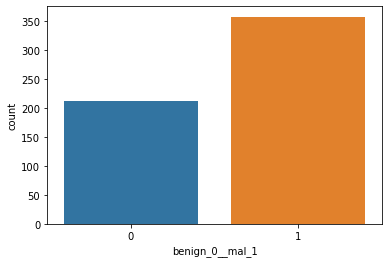This is a good simple example of a classification problem utilizing Keras and Tensorflow. In addition, I’m utilizing early stopping in an attempt to avoid overfitting in the model. You’ll notice this take effect as the model stops training well before the 600 set epochs.

### Keras / Tensorflow Classification – Example

Here we’re going to attempt to utilize Keras/Tensorflow to predict the whether or not an individual has cancer.

The data being used can be seen on my github below:

https://github.com/kaledev/PythonSnippets/blob/master/Datasets/Keras/cancer_classification.csv

#### Data Imports and EDA

In :
```import pandas as pd
import numpy as np
import matplotlib.pyplot as plt
import seaborn as sns
```
In :
```df = pd.read_csv('DATA/cancer_classification.csv')
```

Here we can see the dataset is fairly well balanced in terms of classification of the labels, if the dataset was unbalanced then we might see issues with overfitting.

In :
```sns.countplot(x='benign_0__mal_1',data=df)
```
Out:
`<AxesSubplot:xlabel='benign_0__mal_1', ylabel='count'>`#### Create Models and Predict

In :
```#Set X/y
X = df.drop('benign_0__mal_1', axis=1).values
y = df['benign_0__mal_1'].values
```
In :
```from sklearn.model_selection import train_test_split
```
In :
```X_train, X_test, y_train, y_test = train_test_split(X, y, test_size=0.25, random_state=42)
```

We need to scale the data so all features are in sync

In :
```from sklearn.preprocessing import MinMaxScaler
```
In :
```scaler = MinMaxScaler()
```
In :
```X_train = scaler.fit_transform(X_train)
X_test = scaler.transform(X_test) #Do not fit on testing to avoid overfitting/data leakage
```

Now we can create the Neural Network

In :
```from tensorflow.keras.models import Sequential
from tensorflow.keras.layers import Dense,Dropout
from tensorflow.keras.callbacks import EarlyStopping
```

We’re going to define an early stop here to avoid overfitting and improve accuracy – With Early Stopping, Keras will not run the entirely defined amount of epochs if doing so will cause overfitting.

In :
```early_stop = EarlyStopping(monitor='val_loss',mode='min',verbose=1,patience=25)
```
In :
```X_train.shape
```
Out:
`(426, 30)`

As we see 30 features here, we set the layers to be:
Input: 30
Hidden: 15 (half)
Output: 1

In :
```model = Sequential()

model.add(Dropout(0.5)) #Choose somewhere between 0/1 (1 = 100%) of neurons to turn off

model.add(Dropout(0.5)) #Choose somewhere between 0/1 (1 = 100%) of neurons to turn off

#BINARY CLASSIFICATION MUST BE SIGMOID

#MUST BE binary_crossentropy
```
In :
```model.fit(x=X_train,y=y_train,epochs=600,validation_data=(X_test,y_test),
callbacks=[early_stop])
```
In :
```loss_df = pd.DataFrame(model.history.history)
```
In :
```loss_df.plot()
```
Out:
`<AxesSubplot:>`In :
```predictions = model.predict_classes(X_test)
```
In :
```from sklearn.metrics import classification_report, confusion_matrix
```
In :
```print(classification_report(y_test, predictions))
```
```              precision    recall  f1-score   support

0       0.96      0.98      0.97        54
1       0.99      0.98      0.98        89

accuracy                           0.98       143
macro avg       0.98      0.98      0.98       143
weighted avg       0.98      0.98      0.98       143

```
In :
```print(confusion_matrix(y_test, predictions))
```
```[[53  1]
[ 2 87]]
```

You can see we came out with great results from this model with only 3 incorrectly diagnosed

WordPress conversion from Keras Classification.ipynb by nb2wp v0.3.1